# Item 30: Understand references and reference syntax.

A reference is a scalar value. It can be stored in a scalar variable, or as an element of an array or hash, as is done with numbers and strings. You can think of a reference as a pointer to some other object in Perl. References can point to any kind of object, including other scalars (even references), arrays, hashes, subroutines, and typeglobs.

Aside from a general pointer-like behavior, however, references do not have very much in common with pointers in C or C++. You can only create references to existing objects; you cannot modify them afterward to do something like point to the next element of an array. You can convert references into strings or numbers, but you cannot convert a string or number back into a reference. Although a reference is treated syntactically like any other scalar value, a reference " knows " to what type of object it points. Finally, each reference to a Perl object increments that object's reference count, preventing the object from being scavenged by Perl's garbage collector.

#### Creating references

References can be created in several different ways. The simplest is to use the reference operator \ on a variable:

 ` \$a = 3.1416; \$scalar_ref = \$a; ` A reference to \$a .

The effect of the reference operator is to create a reference pointing at the value of its argument: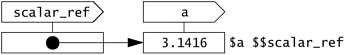The reference operator works on any kind of variable:

 ` \$array_ref = \@a; \$hash_ref = \%a; \$sub_ref = \&a; \$glob_ref = \*a; ` Works on arrays, hashes, subroutines, and even typeglobs.

It also works on array and hash elements, and values:

 ` \$array_el_ref = \$a; \$hash_el_ref = \$a{'hello'}; ` Create refs to array and hash elements. ` \$one_ref = ; \$mode_ref = \oct('0755'); ` \$one_ref is read-only. So is \$mode_ref .

The reference operator works in a very strange way on a list of values, returning a list of references rather than a reference to a list. It decides what to return references to by using a seemingly arbitrary heuristic:

These make a certain amount of sense.

 ` sub val { return 1..3 }; \$ref1 = \(&val); print ref \$ref1, "\n"; \$ref2 = \(val()); print ref \$ref2, " \$\$ref2\n"; (\$ref3) = \(val()); print ref \$ref3, " \$\$ref3\n"; ` The ref operator returns the type of reference. CODE SCALAR 3 SCALAR 1

But these are a little weird.

 ` \$ref4 = \(1..3); print ref \$ref4, " @\$ref4\n"; \$ref5 = \(1, 2, 3); print ref \$ref5, " \$\$ref5\n"; \$ref6 = \(1, 2..3); print ref \$ref6, " @\$ref6\n"; ` ARRAY 1 2 3 SCALAR 3 ARRAY 2 3

You can understand why I recommend that you avoid using the reference operator in front of lists.

The anonymous array constructor [ ] , which looks like an ordinary list except that the contents are enclosed by brackets rather than parentheses, creates an unnamed array in memory and returns a reference to it. The anonymous array constructor is the customary method of creating a reference to a list of items:

 ` \$a_ref = [1..3]; print ref \$a_ref, " @\$a_ref\n"; ` ARRAY 1 2 3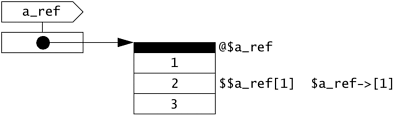The anonymous hash constructor { } , which uses braces rather than brackets, works similarly:

 ` \$h_ref = {}; \$h_ref->{'joe'} = 'bloe'; \$h_ref->{'john'} = 'public'; ` Empty anonymous hash. Add an element. Add another.There are many uses for both anonymous arrays and anonymous hashes. See Items Item 32 and Item 33 for more examples.

A sub definition without a name returns a reference to an anonymous subroutine. References to subroutines are also called code refs :

 ` \$greetings = sub { print "hello, world!\n" }; \$greetings->(); &\$greetings(); ` \$greetings is a code ref. Hello, world. Hello, world. ` \$SIG{INT} = sub { print "not yet--i'm busy\n" }; ` Using an anonymous sub as a signal handler.

References to anonymous subroutines are very useful. They are somewhat like function pointers in C. On the other hand, since anonymous subrou-tines are created dynamically, not statically, they have peculiar properties that are more like something from LISP (see Item 29).

There isn't often much need to construct a reference to an anonymous scalar value, but you can do something like the following if need be:

 ` undef \$s_ref; \$\$s_ref = 2.718; print ref \$s_ref, " \$\$s_ref\n"; ` SCALAR 2.718

This works through "auto-vivification," discussed later in this Item.

Finally, and somewhat mysteriously, you can create references to an undocumented LVALUE type (not exactly the meaning of "lvalue" I give in the Introduction):

 ` \$a = "Testing 1 2 3"; \$lvref = \substr(\$a, 0, 7); \$\$lvref = "Pelham"; print "a = \$a\n"; ` \$lvref is an LVALUE ref. Like assigning to substr ! Pelham 1 2 3

#### Using references

Using the value that a reference points to is called dereferencing . There are several different forms of dereferencing syntax. The "canonical" form of dereferencing syntax is to use a block returning a reference in a place where you could otherwise use a variable or subroutine identifier. Whereas using an identifier would give you the value of the variable with that name, using a block that returns a reference gives you the value to which the reference points:

Canonical syntax for scalar references.

 ` \$a = 1; \$s_ref = \$a; ` \$a is an ordinary scalar. \$s_ref is a reference to the value of \$a . Prints 1 . Works just like a variable. ` print "\${\$s_ref}\n"; \${\$s_ref} += 1; `

Canonical syntax for array references.

 ` @a = 1..5; \$a_ref = \@a; print "@a\n"; print "@{\$a_ref}\n"; push @{\$a_ref}, 6..10; ` \$a_ref is a reference to the value of @a . Prints 1 2 3 4 5 . Also prints 1 2 3 4 5 . Adds elements to @a .

The code inside the block can be arbitrarily complex, so long as the result of the last expression evaluated yields a reference:

 ` \$ref1 = [1..5]; \$ref2 = [6..10]; \$val = \${ if (\$hi) {\$ref2} else {\$ref1} }; print "\$val\n"; ` Returns 3rd element of some array, depending on \$hi . Either 3 or 8 .

If the reference value is contained in a scalar variable, you can dispense with the braces and just use the name of the scalar variable, with the leading \$ , instead. You can use more than one \$ if it's a reference to a reference:

Scalar variable syntax for references.

 ` \$a = 'testing'; \$s_ref = \$a; \$s_ref_ref = \$s_ref; print "\$\$s_ref \$\$\$s_ref_ref\n"; ` testing testing ` \$h_ref = { 'F' => 9, 'Cl' => 17, 'Br' => 35 }; ` Initialize \$h_ref with an anonymous hash. ` print "Elements are ", join ' ', sort(keys %\$h_ref), "\n"; print "F's number: \$\$h_ref{'F'}\n"; ` Elements are Br Cl F F's number: 9

Expressions like \$\$h_ref{'F'} , or the even more awkward equivalent \${\$h_ref}{'F'} , occur frequently. There is a more visually appealing " arrow" syntax that you can use to write subscripts for array and hash references:

 ` \${\$h_ref}{'F'} \$\$h_ref{'F'} \$h_ref->{'F'} ` Canonical syntax. Scalar variable syntax. Arrow syntax.

The arrow syntax also works on code refs:

 ` sub { print sort @_ }->(4,2,5,3,1); ` Prints 12345 .

You can cascade arrows. Furthermore, if the left and right sides of an arrow are both subscripts, you can omit the arrow:

 ` \$student-> = { 'first' => 'joe', 'last' => 'bloe' }; print "\$student->->{'first'}\n"; print "\$student->{'first'}\n"; ` This is a ref to an array of refs to hashes. joe joe same thing.

The data structure in this example looks something like this:Be careful about leaving out too many arrows or braces. For example, if you omit the first arrow, you get an array of hash references, which is different: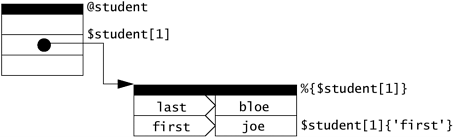There are more examples of complex data structures built from references in the other items in this section.

Finally, as noted earlier, all references, no matter what their type, are handled like ordinary scalarsthey have no special "type" that distinguishes them syntactically from other scalars.  However, a reference value contains information about the type of object to which it points. You can get to this information with the ref operator:

 Mostly, anyway. Don't use a reference as a hash key . Hash keys are always converted to stringswhich are no longer references. If you must use references as hash keys, use the Tie::RefHash module.

 ` \$s_ref = ; print ref \$s_ref, "\n"; \$c_ref = sub { 'code!' }; print ref \$c_ref, "\n"; ` SCALAR CODE

The ref operator works differently on blessed objects (see Item 49).

#### Auto-vivification

If you use a scalar lvalue with an undefined value as if it were a reference to another object, Perl will automatically create an object of the appropriate type for you and make that scalar a reference to that object. This is called auto-vivification . For example, the following code creates an array of four elements and makes \$ref a reference to it:

 ` undef \$ref; \$ref-> = 'four'; ` \$ref is now empty. \$ref springs into being!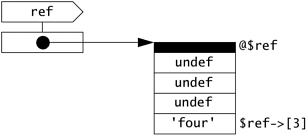A longer example of auto-vivification is discussed in Item 31.

#### Soft references

If you dereference a string value, Perl will return the value of the variable with the name given in the string. The variable will be created if necessary. This is called a soft reference .

 ` \$str = 'pi'; \${\$str} = 3.1416; print "pi = \$pi\n"; ` pi = 3.1416 ` \${'e' . 'e'} = 2.7183; print "ee = \$ee\n"; ` ee = 2.7183

Such a variable name does not have to be a legal identifier:

 ` \${' '} = 'space'; \${ } = 'space'; ` The space variable. ILLEGAL now; used to be same as \${' '} . The space space variable. The null variable. ` \${' '} = 'two space'; \${" \${' '} = 'two space'; \${"\0"} = 'null'; "} = 'null'; `

Note that soft references have nothing to do with reference counts (see Item 34). Only ordinary "hard" references increment reference counts. Turning on strict refs disables soft references (see Item 36)this is often a good idea.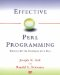Effective Perl Programming: Writing Better Programs with Perl
ISBN: 0201419750
EAN: 2147483647
Year: 1996
Pages: 116

Similar book on Amazon

flylib.com © 2008-2017.
If you may any questions please contact us: flylib@qtcs.net